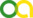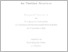Ciolan, Emil-Alexandru (2020). Asymptotics, Equidistribution and Inequalities for Partition Functions. PhD thesis, Universität zu Köln.Preview
PDF
DissertationCiolanFinalA5.pdf - Published Version
Bereitstellung unter der CC-Lizenz: Creative Commons Attribution.

## Abstract

This thesis consists of three research projects on asymptotics, equidistribution properties and inequalities for partition and overpartition functions. We start by proving that the number of partitions into squares with an even number of parts is asymptotically equal to that of partitions into squares with an odd number of parts. We further show that, for $n$ large enough, the two quantities are different, and that which of the two is bigger depends on the parity of $n.$ By doing so, we answer a conjecture formulated by Bringmann and Mahlburg (2012). We continue by placing this problem in a broader context and by proving that the same results are true for partitions into any powers. For this, we invoke an estimate on Gauss sums found by Banks and Shparlinski (2015) using the effective lower bounds on center density from the sphere packing problem established by Cohn and Elkies (2003). Finally, we compute asymptotics for the coefficients of an infinite class of overpartition rank generating functions, and we show that $\overline{N}(a,c,n),$ the number of overpartitions of $n$ with rank congruent to $a$ modulo $c,$ is equidistributed with respect to $0\le a< c,$ as $n\to\infty,$ for any $c\ge2.$ In addition, we prove some inequalities between ranks of overpartitions recently conjectured by Ji, Zhang and Zhao (2018), and Wei and Zhang (2018).

Item Type: Thesis (PhD thesis)
Creators:
CreatorsEmailORCIDORCID Put Code
Ciolan, Emil-Alexandruaciolan@math.uni-koeln.deUNSPECIFIEDUNSPECIFIED
URN: urn:nbn:de:hbz:38-115582
Date: 21 July 2020
Language: English
Faculty: Faculty of Mathematics and Natural Sciences
Divisions: Faculty of Mathematics and Natural Sciences > Department of Mathematics and Computer Science > Mathematical Institute
Subjects: Mathematics
Uncontrolled Keywords:
KeywordsLanguage
Asymptotics, circle method, equidistribution, Gauss sums, inequalities, Kloosterman sums, overpartitions, partitions, ranksEnglish
Date of oral exam: 23 June 2020
Referee:
Bringmann, KathrinProf. Dr.
Zwegers, SanderProf. Dr.
Refereed: Yes
URI: http://kups.ub.uni-koeln.de/id/eprint/11558View Item